## How to Calculate and Solve for Gravity Anomaly for an Infinitely Long Cylinder | Gravity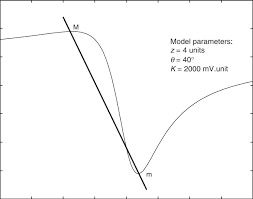The image above represents gravity anomaly for an infinitely long cylinder.

To compute for gravity anomaly for an infinitely long cylinder, five essential parameters are needed and these parameters are Gravitational Constant (G), Anomalous Density (Δρ), Radius of Cylinder (b), Depth Buried (d) and Horizontal Distance (x).

The formula for calculating gravity anomaly for an infinitely long cylinder:

δgx = GΔρ2πb²d / x² + d²

Where:

δgx = Gravity Anomaly for an Infinitely Long Cylinder
G = Gravitational Constant
Δρ = Anomalous Density
d = Depth Buried
x = Horizontal Distance

Let’s solve an example;
Find the gravity anomaly for an infinitely long cylinder when the gravitational constant is 6.67E-11, the anomalous density is 9, the radius of cylinder is 11, the depth buried is 8 and the horizontal distance is 10.

This implies that;

G = Gravitational Constant = 6.67E-11
Δρ = Anomalous Density = 9
b = Radius of Cylinder = 11
d = Depth Buried = 8
x = Horizontal Distance = 10

δgx = GΔρ2πb²d / x² + d²
δgx = 6.67e-11(9)2π(11)²(8) / (10)² + (8)²
δgx = 6.67e-11(9)2π(121)(8) / (100) + (64)
δgx = 6.67e-11(9)(6082.123) / 164
δgx = 0.000003651098 / 164
δgx = 2.226e-8

Therefore, the gravity anomaly for an infinitely long cylinder is 2.226e-8 mGal.

## How to Calculate and Solve for Free Air Anomaly | Gravity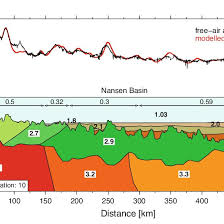The image above represents free air anomaly.

To compute for free air anomaly, four essential parameters are needed and these parameters are Observed or Measured Gravity Value (gobs), Reference Value of Gravity (g(λ)), Elevation (h) and Radius of the Earth (R).

The formula for calculating free air anomaly:

δgF = gobs – g(λ) [1 – 2h/R]

Where:
δgF = Free Air Anomaly
gobs = Observed or Measured Gravity Value
g(λ) = Reference Value of Gravity
h = Elevation
R = Radius of the Earth

Let’s solve an example;
Find the free air anomaly when the observed or measured gravity value is 9, reference value of gravity is 10, elevation is 16 and radius of the earth is 4.

This implies that;

gobs = Observed or Measured Gravity Value = 9
g(λ) = Reference Value of Gravity = 10
h = Elevation = 16
R = Radius of the Earth = 4

δgF = gobs – g(λ) [1 – 2h/R]
δgF = 9 – 10 [1 – 2(16)/4]
δgF = 9 – 10 [1 – 32/4]
δgF = 9 – 10 [1 – 8]
δgF = 9 – 10 [-7]
δgF = 9 – -70
δgF = 79

Therefore, the free air anomaly is 79 Gal.

## How to Calculate and Solve for Gravitational Acceleration | Gravity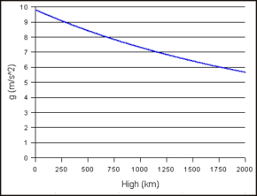The image above represents gravitational acceleration.

To compute for gravitational acceleration, three essential parameters are needed and these parameters are Gravitational Constant (G), Mass of the Earth (mEand Radius of the Earth (R).

The formula for calculating gravitational acceleration:

a = GmE /

Where:

a = Gravitational Acceleration
G = Gravitational Constant
mE = Mass of the Earth
R = Radius of the Earth

Let’s solve an example;
Find the Gravitational acceleration when the gravitational constant is 6.67E-11, the mass of the earth is 12 and the radius of the earth is 21.

This implies that;

G = Gravitational Constant = 6.67E-11
mE = Mass of the Earth = 12
R = Radius of the Earth = 21

a = GmE /
a = (6.67e-11)(12) / (21)²
a = 8.004e-10 / 441
a = 1.81e-12

Therefore, the gravitational acceleration is 1.81e-12 m/s.

## How to Calculate and Solve for Intensity of Induced Magnetization (K, H) | Magnetic Field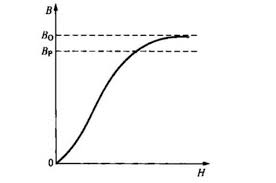The image above represents intensity of induced magnetization.

To compute for intensity of induced magnetization, two essential parameters are needed and these parameters are Magnetic Susceptibility (K) and Magnetizing Force of Inducing Field (H).

The formula for calculating intensity induced magnetization:

J = KH

Where:

J = Intensity of Induced Magnetization
K = Magnetic Susceptibility
H = Magnetizing Force of Inducing Field

Let’s solve an example;
Find the intensity induced magnetization when the magnetic susceptibility is 14 and the magnetizing force of inducing field is 24.

This implies that;

K = Magnetic Susceptibility = 14
H = Magnetizing Force of Inducing Field = 24

J = KH
J = (14)(24)
J = 336

Therefore, the intensity induced magnetization is 336.

Calculating the Magnetic Susceptibility when the Intensity Induced Magnetization and the Magnetizing Force of Induced Field is Given.

K = J / H

Where;

K = Magnetic Susceptibility
J = Intensity Induced Magnetization
H = Magnetizing force of Induced Field

Let’s solve an example;
Find the magnetic susceptibility when the intensity induced magnetization is 40 and the magnetizing force of Induced Field is 4.

This implies that;

J = Intensity Induced Magnetization = 40
H = Magnetizing force of Induced Field = 4

K = J / H
K = 40 / 4
K = 10

Therefore, the magnetic susceptibility is 10.

## How to Calculate and Solve for Magnetic Flux | Magnetic Field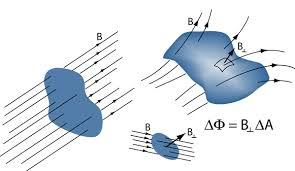The image above represents magnetic flux.

To compute for magnetic flux, two essential parameters are needed and these parameters are Area (A) and Total Magnetic Field (B).

The formula for calculating magnetic flux:

φ = B.A

Where:

φ = Magnetic Flux
A = Area
B = Total Magnetic Field

Let’s solve an example;
Find the magnetic flux with a given area of 32 and a total magnetic field of 18.

This implies that;

A = Area = 32
B = Total Magnetic Field = 18

φ = B.A
φ = (18)(32)
φ = 576

Therefore, the magnetic flux is 576 Wb.

Calculating the Area when the Magnetic Flux and the Total Magnetic Field is Given.

A = φ / B

Where;

A = Area
φ = Magnetic Flux
B = Total Magnetic Field

Let’s solve an example;
Find the area when the magnetic flux is 40 and the total magnetic field is 20.

This implies that;

φ = Magnetic Flux = 40
B = Total Magnetic Field = 20

A = φ / B
A = 40 / 20
A = 2

Therefore, the area is 2.

## How to Calculate and Solve for Intensity of Induced Magnetization | Magnetic FieldThe image above represents intensity induced magnetization.

To compute for intensity induced magnetization, three essential parameters are needed and these parameters are Magnetic Moment (M), Length (L) and Cross-Sectional Area (A).

The formula for calculating intensity induced magnetization:

J = M / LA

Where:

J = Intensity of Induced Magnetization
M = Magnetic Moment
L = Length
A = Cross-Sectional Area

Let’s solve an example;
Find the intensity induced magnetization when the magnetic moment is 20, the length is 16 and the cross-sectional area is 25.

This implies that;

M = Magnetic Moment = 20
L = Length = 16
A = Cross-Sectional Area = 25

J = M / LA
J = 20 / (16)(25)
J = 20 / 400
J = 0.05

Therefore, the intensity induced magnetization is 0.05.

Calculating the Magnetic Moment when the Intensity Induced Magnetization, the Length and the Cross-Sectional Area is Given.

M = J x LA

Where;

M = Magnetic Moment
J = Intensity Induced Magnetization
L = Length
A = Cross-Sectional Area

Let’s solve an example;
Find the Magnetic Moment when the intensity induced magnetization is 20, the length is 12 and the cross-sectional area is 5.

This implies that;

J = Intensity Induced Magnetization = 20
L = Length = 12
A = Cross-Sectional Area = 5

M = J x LA
M = 20 x (12)(5)
M = 20 x 60
M = 1200

Therefore, the magnetic moment is 1200.

## How to Calculate and Solve for Magnetic Potential | Magnetic Field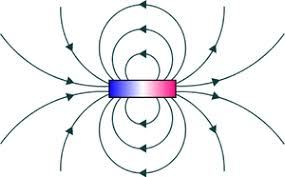The image above represents magnetic potential.

To compute for magnetic potential, four essential parameters are needed and these parameters are Magnetic Permeability of Vacuum (μo), Strength of Magnetic Pole (m), Relative Magnetic Permeability (μRand Seperating Distance (r).

The formula for calculating magnetic potential:

v = μom / 4πμRr

Where:

v = Magnetic Potential
μo = Magnetic Permeability of Vacuum
m = Strength of Magnetic Pole
μR = Relative Magnetic Permeability
r = Separating Distance

Let’s solve an example;
Find the magnetic potential when the magnetic permeability of vaccum is 1.2566371E-6, the strength of magnetic pole is 10, the relative magnetic permeability is 22 and seperating distance is 18.

This implies that;

μo = Magnetic Permeability of Vacuum = 1.2566371E-6
m = Strength of Magnetic Pole = 10
μR = Relative Magnetic Permeability = 22
r = Separating Distance = 18

v = μom / 4πμRr
v = (0.0000012566371)(10) / 4π(22)(18)
v = 0.000012566371000000001 / 4976.282
v = 2.52e-9

Therefore, the magnetic potential is 2.52e-9.

## How to Calculate and Solve for Magnetic Field | Magnetic Field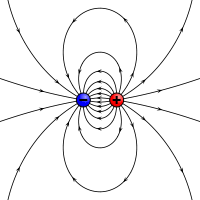The image above represents magnetic field.

To compute for magnetic field, four essential parameters are needed and these parameters are Magnetic Permeability of Vacuum (μo), Strength of Magnetic Pole (m), Relative Magnetic Permeability (μRand Seperating Distance (r).

The formula for calculating magentic field:

B = μom / 4πμRr²

Where:

B = Magnetic Field
μo = Magnetic Permeability of Vacuum
m = Strength of Magnetic Pole
μR = Relative Magnetic Permeability
r = Separating Distance

Let’s solve an example;
Find the magnetic field when the magnetic permeability of vaccum is 1.2566371E-6, strength of magnetic pole is 11, relative magnetic permeability is 9 and the seperating distance is 12.

This implies that;

μo = Magnetic Permeability of Vacuum = 1.2566371E-6
m = Strength of Magnetic Pole = 11
μR = Relative Magnetic Permeability = 9
r = Separating Distance = 12

B = μom / 4πμRr²
B = (0.0000012566371)(11) / 4π(9)(12)²
B = 0.00001382 / 4π(9)(144)
B = 0.00001382 / (16286.0163)
B = 8.487e-10

Therefore, the magnetic field is 8.487e-10.

## How to Calculate and Solve for Magnetic Moment of a Dipole | Magnetic Field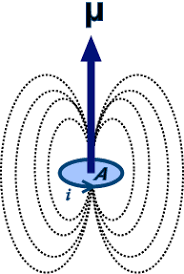The image above represents magnetic moment of a diople.

To compute for magnetic moment of a diople, two essential parameters are needed and these parameters are Magnetic Pole Strength (m) and Distance Seperating Poles (l).

The formula for calculating magnetic moment of a diople:

M = ml

Where;

M = Magnetic Moment of a Diople
m = Magnetic Pole Strength
l = Distance Seperating Poles

Let’s solve an example;
Find the magnetic moment of a diople when the magentic pole strength is 21 and the distance seperating poles is 13.

This implies that;

m = Magnetic Pole Strength = 21
l = Distance Seperating Poles = 13

M = ml
M = 21 x 13
M = 273

Therefore, the magnetic moment of a diople is 273.

Calculating the Magnetic Pole Strength when the Magnetic Moment of a Diople and the Distance Seperating Poles is Given.

m = M / l

Where;

m = Magnetic Pole Strength
M = Magnetic Moment of a Diople
l = Distance Seperating Poles

Let’s solve an example;
Find the magnetic pole strength when the magnetic moment of a diople is 30 and the distance seperating poles is 5.

This implies that;

M = Magnetic Moment of a Diople = 30
l = Distance Seperating Poles = 5

m = M / l
m = 30 / 5
m = 6

Therefore, the magnetic pole strength is 6.

## How to Calculate and Solve for Force of Attraction or Repulsion | Magnetic Field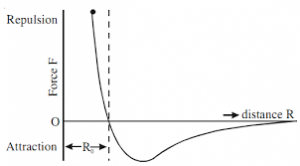The image above represents force of attraction or repulsion.

To compute for force of attraction or repulsion, five essential parameters are needed and these parameters are Magnetic Permeability of Vacuum (μo), Strength of Magnetic Pole 1 (m1), Strength of Magnetic Pole 2 (m2), Distance Seperating the Poles (r) and Relative Magnetic Permeability (μR).

The formula for calculating force of attraction or repulsion:

F = μom1m2 / 4πμRr²

Where:

F = Force of Attraction or Repulsion
μo = Magnetic Permeability of Vacuum
m1 = Strength of Magnetic Pole 1
m2 = Strength of Magnetic Pole 2
r = Distance Separating the Poles
μR = Relative Magnetic Permeability

Let’s solve an example;
Find the force of attraction or repulsion when the magnetic permeability of vaccum is 1.2566371E-6, strength of magnetic pole 1 is 10, strength of magnetic pole 2 is 12, distance seperating the poles is 8 and the relative magnetic permeability is 14.

This implies that;

μo = Magnetic Permeability of Vacuum = 1.2566371E-6
m1 = Strength of Magnetic Pole 1 = 10
m2 = Strength of Magnetic Pole 2 = 12
r = Distance Separating the Poles = 8
μR = Relative Magnetic Permeability = 14

F = μom1m2 / 4πμRr²
F = 0.0000012566371(10)(12) / 4π(14)(8)²
F = 0.000150796452 / 4π(14)(64)
F = 0.000150796452 / 11259.468
F = 1.339e-8

Therefore, the force of attraction or repulsion is 1.339e-8.